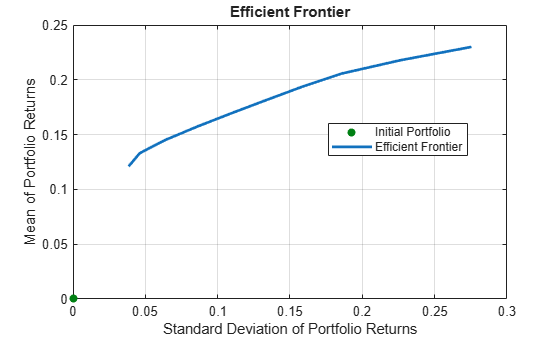# getOneWayTurnover

Obtain one-way turnover constraints from portfolio object

## Syntax

``````[BuyTurnover,SellTurnover] = getOneWayTurnover(obj)``````

## Description

Use the `getOneWayTirnover` function with a `Portfolio`, `PortfolioCVaR`, or `PortfolioMAD` object to obtain one-way turnover constraints from portfolio objects.

For details on the respective workflows when using these different objects, see Portfolio Object Workflow, PortfolioCVaR Object Workflow, and PortfolioMAD Object Workflow.

example

``````[BuyTurnover,SellTurnover] = getOneWayTurnover(obj)``` obtain one-way turnover constraints from portfolio objects.```

## Examples

collapse all

Set one-way turnover costs.

```p = Portfolio('AssetMean',[0.1, 0.2, 0.15], 'AssetCovar',... [ 0.005, -0.010, 0.004; -0.010, 0.040, -0.002; 0.004, -0.002, 0.023]); p = setBudget(p, 1, 1); p = setOneWayTurnover(p, 1.3, 0.3, 0); %130-30 portfolio plotFrontier(p);```Obtain one-way turnover costs.

`[BuyTurnover,SellTurnover] = getOneWayTurnover(p)`
```BuyTurnover = 1.3000 ```
```SellTurnover = 0.3000 ```

Set one-way turnover costs and obtain the buy and sell turnover values.

```m = [ 0.05; 0.1; 0.12; 0.18 ]; C = [ 0.0064 0.00408 0.00192 0; 0.00408 0.0289 0.0204 0.0119; 0.00192 0.0204 0.0576 0.0336; 0 0.0119 0.0336 0.1225 ]; m = m/12; C = C/12; AssetScenarios = mvnrnd(m, C, 20000); p = PortfolioCVaR; p = setScenarios(p, AssetScenarios); p = setDefaultConstraints(p); p = setProbabilityLevel(p, 0.95); p = setBudget(p, 1, 1); p = setOneWayTurnover(p, 1.3, 0.3, 0); %130-30 portfolio [BuyTurnover,SellTurnover] = getOneWayTurnover(p)```
```BuyTurnover = 1.3000 ```
```SellTurnover = 0.3000 ```

Set one-way turnover costs and obtain the buy and sell turnover values.

```m = [ 0.05; 0.1; 0.12; 0.18 ]; C = [ 0.0064 0.00408 0.00192 0; 0.00408 0.0289 0.0204 0.0119; 0.00192 0.0204 0.0576 0.0336; 0 0.0119 0.0336 0.1225 ]; m = m/12; C = C/12; AssetScenarios = mvnrnd(m, C, 20000); p = PortfolioMAD; p = setScenarios(p, AssetScenarios); p = setDefaultConstraints(p); p = setBudget(p, 1, 1); p = setOneWayTurnover(p, 1.3, 0.3, 0); %130-30 portfolio [BuyTurnover,SellTurnover] = getOneWayTurnover(p)```
```BuyTurnover = 1.3000 ```
```SellTurnover = 0.3000 ```

## Input Arguments

collapse all

Object for portfolio, specified using `Portfolio`, `PortfolioCVaR`, or `PortfolioMAD` object. For more information on creating a portfolio object, see

Data Types: `object`

## Output Arguments

collapse all

Turnover constraint on purchases, returned as a scalar for a `Portfolio`, `PortfolioCVaR`, or `PortfolioMAD` input object (`obj`).

Turnover constraint on sales, returned as a scalar for a `Portfolio`, `PortfolioCVaR`, or `PortfolioMAD` input object (`obj`).

collapse all

### One-way Turnover Constraint

One-way turnover constraints ensure that estimated optimal portfolios differ from an initial portfolio by no more than specified amounts according to whether the differences are purchases or sales.

The constraints take the form

`${1}^{T}\mathrm{max}\left\{0,x-{x}_{0}\right\}\le {\tau }_{B}$`

`${1}^{T}\mathrm{max}\left\{0,{x}_{0}-x\right\}\le {\tau }_{S}$`

with

• `x` — The portfolio (NumAssets vector)

• `x0` — Initial portfolio (NumAssets vector)

• τB — Upper-bound for turnover constraint on purchases (scalar)

• τS — Upper-bound for turnover constraint on sales (scalar)

Specify one-way turnover constraints using the following properties in a supported portfolio object: `BuyTurnover` for τB, `SellTurnover` for τS, and `InitPort` for `x0`.

Note

The average turnover constraint (which is set using `setTurnover`) is not just the combination of the one-way turnover constraints with the same value for the constraint.

## Tips

You can also use dot notation to get the one-way turnover constraint for portfolio objects.

`[BuyTurnover,SellTurnover] = obj.getOneWayTurnover`

Introduced in R2011a